Next: Normalization. Up: Probabilistic ancestral sequences and Previous: Independence of the position

# Probabilistic Dynamic Programming and Multiple Alignments

The standard DP algorithm for aligning two sequences [15,18,10], requires that we estimate a cost of matching any two individual positions. This is done by the method described in the introduction or by completely empirical cost/similarity functions which assign some value to the alignment of each pair of amino acids. The DP algorithm for aligning sequences can be viewed as an algorithm which takes as parameter such a cost/similarity function,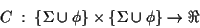and produces an alignment which maximizes the sum of the Cfunction applied to all aligned pairs1.

We will use DP over partially built multiple alignments and the C function will be derived from the probability of an EC. We call this aligning algorithm probabilistic dynamic programming.

More precisely, our DP algorithm works over two partial multiple alignments. A partial multiple alignment is a multiple alignment of all the sequences of a subtree of the EPT. Hence a partial multiple alignment is identified by an internal node and a direction in the EPT. E.g. node Y has as descendants the sequence 3 and the node W; node W has sequences 1 and 2 as descendants. The partial multiple alignment for node Y is:

  1     FKQCCWNSLP____RGLSNVALVYQEFMAKCRGESENLQLVTALVINLPSMA
2   SMFRQCIWNSLS____HGLPETAPIYQPLKARCRGVSENLQLVTEIIINLPTLC
3   SLWCQCIKASLPLKVIRGTPEVAPLYDQLEQVCRSENQ____VSEIVAKFASLC

For each subtree X and its partial multiple alignment we also compute the TX and SX vectors. The C function for this algorithm will be derived from the probability of alignment of two slices of a multiple alignment under the same ancestor. In essence, this is exactly the same idea as in Dayhoff , extended so that we can compute the probabilities of alignments not just with two descendant sequences, but with two subtrees of descendants.

The C function is the logarithm of the quotient of probabilities, or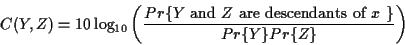(The factor 10 is retained for purely historical reasons, it was used first by Dayhoff et al. Any constant factor can be used without any material effect.) Using the T and S vectors this becomes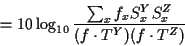At this point, we are using DP to align the two subtrees rooted at Y and Z. Hence we still do not have a correspondence between each position of Y and each position of Z. That is why we have to use the sum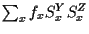in C. Once that we have found the alignment of Y and Z, then we can compute TX and SX for the node we have just joined, the parent of Y and Z.

The cost of each computation of C ismultiplications. The cost of one probabilistic DP alignment is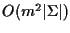and the cost of the entire process (including the computation of S and T) is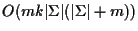.

The alignment at the root of the EPT is the multiple alignment of all the sequences. Notice that for two sequences, this algorithm coincides exactly with DP using Dayhoff matrices, so this presents a continuous generalization of the 2-sequence algorithm.Next: Normalization. Up: Probabilistic ancestral sequences and Previous: Independence of the position
Gaston Gonnet
1998-07-14